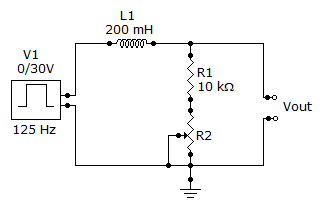# Online Electrical Engineering Test - Electrical Engineering Test 4Loading Test...

Instruction:

• This is a FREE online test. DO NOT pay money to anyone to attend this test.
• Total number of questions : 20.
• Time alloted : 30 minutes.
• Each question carry 1 mark, no negative marks.
• DO NOT refresh the page.
• All the best :-).

1.

When these numbers are multiplied, (6 × 103) (5 × 105), the result is

A.
 3 × 108B.
 30 × 108C.
 300 × 109D.
 3,000 × 1072.

A material that does not allow current under normal conditions is a(n)

A.
 insulatorB.
 conductorC.
 semiconductorD.
 valence3.

A circuit breaker is a

A.
 fuseB.
 switchC.
 resettable protective deviceD.
 resistor4.

A current of 200 µA through a 6.8 kresistor produces a voltage drop of

A.
 34.4 VB.
 340 VC.
 13.6 VD.
 1.36 V5.

A 6 V battery is connected to a 300load. Under these conditions, it is rated at 40 Ah. How long can it supply current to the load?

A.
 1 hB.
 200 hC.
 2,000 hD.
 10 h6.

A 68resistor is connected across the terminals of a 3 V battery. The power dissipation of the resistor is

A.
 132 mWB.
 13.2 mWC.
 22.6 mWD.
 226 mW7.

The unit for reluctance is

A.
 teslaB.
 At/WbC.
 At/mD.
 Wb8.

The conductive loop on the rotor of a simple two-pole, single-phase generator rotates at a rate of 400 rps. The frequency of the induced output voltage is

A.
 40 HzB.
 100 HzC.
 400 HzD.
 indeterminable9.

A capacitor and a resistor are connected in series to a sine wave generator. The frequency is set so that the capacitive reactance is equal to the resistance and, thus, an equal amount of voltage appears across each component. If the frequency is increased

A.
 VR > VCB.
 VC > VRC.
 VR = VCD.
 VR and VC = 010.

In Question 6, the capacitor will reach full charge in a time equal to approximately

A.
 6 RCB.
 5 RCC.
 RCD.
 none of the above11.

When the current through an inductor decreases, the amount of energy stored in the electromagnetic field

A.
 increasesB.
 decreasesC.
 remains the sameD.
 doubles12.

How many primary volts must be applied to a transformer with a turns ratio of 0.1 to obtain a secondary voltage of 9 V?

A.
 9 VB.
 90 VC.
 900 VD.
 0.9 VExplanation:

We know that, V2/V1 = N2/N1.

Given V2 = 9v, N2/N1 = 0.1

Therefore, 9v/V1 = 0.1

V1 = 9v/0.1 = 90v.

For more detail: Step Down Transformer - Circuit with Simulation.

13.

Referring to Problem 21, what is the phase angle for the circuit?

A.
 7.1°B.
 71.5°C.
 1.8°D.
 18.4°14.

A 470resistor and a 0.2F capacitor are in parallel across a 2.5 kHz ac source. The admittance, Y, in rectangular form, is

A.
 212B.
 2.12 mS + j3.14 mSC.
 3.14 mS + j2.12 mSD.
 318.315.

The polar form of a complex number consists of a real part and a j part.

A.
 TrueB.
 False16.

A 470resistor and a coil with 125inductive reactance are in parallel. Both components are across a 15 V ac voltage source. Current through the inductor is

A.
 152 mAB.
 32 mAC.
 12 mAD.
 120 mA17.

The power factor indicates how much of the apparent power is reactive power.

A.
 TrueB.
 False18.

Referring to Problem 12, the voltage across the capacitor is

A.
 7.8 VB.
 780 mVC.
 5.4 VD.
 540 V19.

Referring this circuit, determine the maximum output voltage when a single pulse is applied as shown. The total resistance is 60.A.
 2.73 VB.
 27.33 VC.
 30 VD.
 2.67 V20.

Referring to Problem 3, power consumption is

A.
 16 WB.
 160 mWC.
 160 WD.
 3,459 W